Thank you for visiting nature.com. You are using a browser version with limited support for CSS. To obtain the best experience, we recommend you use a more up to date browser (or turn off compatibility mode in Internet Explorer). In the meantime, to ensure continued support, we are displaying the site without styles and JavaScript.

# Magnetic Metamaterial Superlens for Increased Range Wireless Power Transfer

## Abstract

The ability to wirelessly power electrical devices is becoming of greater urgency as a component of energy conservation and sustainability efforts. Due to health and safety concerns, most wireless power transfer (WPT) schemes utilize very low frequency, quasi-static, magnetic fields; power transfer occurs via magneto-inductive (MI) coupling between conducting loops serving as transmitter and receiver. At the “long range” regime – referring to distances larger than the diameter of the largest loop – WPT efficiency in free space falls off as (1/d)6; power loss quickly approaches 100% and limits practical implementations of WPT to relatively tight distances between power source and device. A “superlens”, however, can concentrate the magnetic near fields of a source. Here, we demonstrate the impact of a magnetic metamaterial (MM) superlens on long-range near-field WPT, quantitatively confirming in simulation and measurement at 13–16 MHz the conditions under which the superlens can enhance power transfer efficiency compared to the lens-less free-space system.

## Introduction

The superlens, which can refocus not only propagating far-field waves but also non-propagating, near-field waves, has been one of the more provocative concepts to emerge from the field of metamaterials1. A superlens comprises a medium whose electric permittivity ε and magnetic permeability μ both take on the value of −1. The superlens structure offers a means of controlling and manipulating the near-fields that would otherwise decay rapidly away from a source. Initially, the superlens was proposed in the context of optics, where its use was suggested as a means of forming an image with resolution greater than that implied by the diffraction limit2,3. The superlens functions via the excitation of magnetic and electric surface modes that couple to the near fields of an object placed on one side of the slab, subsequently bringing them to a focus on the opposite side. Since electricity and magnetism are nearly decoupled in the near field, it was realized early on that a superlens with either ε = −1 or μ = −1 could focus the near field of electric or magnetic sources, respectively. Imaging with a superlens has been demonstrated at visible and infrared wavelengths using thin layers of materials such as silver or silicon carbide whose dielectric functions take the value of ε = −1 at particular wavelengths4.

At low frequencies, where magnetism is much more prevalent, superlenses based on structured metamaterials characterized as artificial magnetic permeability media have been pursued for a variety of applications, including as flux guides to enhance resolution in magnetic resonance imaging5,6. More recently, as interest in WPT schemes has risen, the use of superlenses and other metamaterial-based components to enhance transfer efficiency has been suggested7. At the low frequencies typical of inductive WPT schemes, the excitation wavelength exceeds 10 m, whereas the dimension of the coils and loops is on the decimeter or centimeter scale. Thus, the loops can be initially approximated as magnetic dipoles as a route to gaining an intuitive understanding of the limits and behavior of the WPT system.

A general treatment of a magneto-inductive WPT system was carried out by Kurs et al., who made use of coupled-mode theory to calculate the expected efficiency of two self-resonant coils separated by a distance d in free space8. In that work and the subsequent analysis of Urzhumov et al.9, power transfer efficiency is defined as the power dissipated in a load placed on the receiver coil (Rx) divided by the total power dissipated in the transmitter (Tx) and receiver circuits, as well as in any intermediate “relay systems” (such as a repeater coil, lens or metamaterial layer):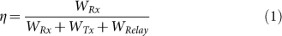Once the Rx and Tx circuits and an optional relay system are specified, power dissipation rates can be calculated using the coupled mode theory for arbitrary Rx and Tx configurations8, or the simplified coupled-dipole formalism for small resonant coils9. Coupled mode theory treats the Rx and Tx coils as resonators whose interaction is indicated by a mode coupling coefficient, κ, whereas the coupled-dipole theory uses the conventional notion of mutual inductance, L21. Both theories predict the same long-range behavior for the WPT efficiency (1), namely, η ~ d−6. The physical origin of this power law is due to the 1/d3 dependence of magnetic field in the near field of a magnetic dipole source. The overall system efficiency in magneto-inductive schemes is therefore inherently limited by the divergence of magnetic flux in free space, because power transfer is ultimately related to the amount of flux from the first coil that can be captured in the “aperture” of the second coil. Given that any coil or loop will behave roughly as a magnetic dipole, it is unlikely that any redesign or engineering of the coils can possibly enhance WPT efficiency of any system that is already optimally impedance-matched.

If the efficiency of a resonant WPT system is to be improved, a means must be found to recapture and refocus the otherwise divergent magnetic flux. Traditional lenses based on conventional materials only focus the far-fields and therefore are irrelevant to this application. Near-field lenses of several configurations10,11,12 have been proposed; however those configurations provide partial field focusing with efficiency significantly lower than the perfect focusing promised by the superlens13.

The use of a near-field superlens in conjunction with resonant power transfer was first considered by Wang et al14. Earlier, similar metamaterial lenses were proposed for magnetic resonance imaging applications15,16. Examining both isotropic14 as well as anisotropic17 versions of the negative-permeability medium, these works provided significant numerical evidence and some experimental evidence, of enhanced efficiency. Here, we address the question raised by the studies in Ref. 14,17, as to whether metamaterial superlenses can improve WPT efficiency (and conversely, reduce power loss) in the long-range near-field transfer regime.

Theoretically, a positive answer to this important question was given in Ref. 9. Figure 1 is based on the analytical solutions from Ref. 9 and it shows that a metamaterial with finite and realistic resistive/magnetic loss can help deliver magnetic flux and generate alternating current in a resonant Rx circuit, leading to overall improvement in WPT efficiency. This performance boost was predicted in the long-range, high-load transfer regime, where both the transfer distance d and the resistive load in the receive circuit exceed certain thresholds. This Report presents experimental evidence that long-range WPT efficiency using a MM slab can exceed the maximum efficiency obtainable, ceteris paribus, in free space.

## Results

### Negative-permeability superlens design

Geometrically, the superlens is perhaps the simplest possible configuration for near-field focusing: it consists of a uniform layer of isotropic, negative permeability. This property simplifies the superlens design, which could be as simple as a double- or triple-periodic arrangement of identical unit cells. In addition, the superlens is translationally invariant, at least in the large aperture limit, which reduces the need for fine mechanical alignment of the WPT system relative to the lens. Figure 2 illustrates the geometry of our superlens implementation; the unit cell geometry, design, homogenization and effective permeability are detailed in Methods. The major challenge for designing negative-permeability metamaterials at relatively low frequencies (MHz regime and lower) is the design of sufficiently low loss, strong magnetic dipole resonators of deeply sub-wavelength dimensions. Here, we have opted for unit cells of size ~ 2 cm and achieved sufficiently large inductance by using multi-turn planar coils18.

### Enhancement of Magneto-inductive coupling with finite aperture superlens

Once a metamaterial layer with desired effective permeability is designed, we can characterize its effect on the coupling between two magnetic dipoles. We approximate a finite-aperture slab as a disk of the same diameter, which enables highly efficient axisymmetric description along the lines of Ref. 9,17 in a 2D rotationally-symmetric geometry modeled in COMSOL Multiphysics. We use the retrieved permeability components of the metamaterial slab, each fitted to a Lorentzian resonance shape, as the components of the slab diagonal permeability tensor μ = [μT μT μN] (assuming all off-diagonal elements are zero and relative permittivity is εr = +1). The transmitting magnetic dipole is simulated as a current loop of radius r = 1 cm carrying a fixed current I = 1A. We define the magnetic field transmission coefficient as T = |HRx/HTx|2, where HTx = I/2r is used to approximate the total field radiating from the Tx loop and HRx is the field measured at a point on the axis-of-revolution a distance d away from the loop. It is convenient to define transmission enhancement factor due to the slab, using the ratio of transmission coefficients with and without the slab G = Tslab/Tvac.

Since the simulated coil is small relative to the slab and can be approximated as a dipole, we use the simulation results to validate the analytical solutions for the inductance between two dipoles with and without the MM slab (and, respectively) as expressed in Ref. 9. In Figure 3 we plot the simulated and analytical enhancement factors (the latter, defined as, we evaluate numerically with Mathematica). Results indicate that the slab enhances the field transmission coefficient T by roughly a factor of five over an appreciable frequency region. In addition, we observe a striking agreement between simulation and analytical results as a function of frequency.

### Measurements of enhancement with non-resonant coils

To experimentally verify the effect of the metamaterial “superlens” on WPT between two coils, we begin by constructing a slab from the fabricated MM. To assemble a 1-layer slab, we first designed the x- and y-oriented MM elements in rows with opposite-facing slits, which can be assembled into a wine-crate pattern, as shown in Figure 2A. The z-oriented resonators were fabricated across a single sheet, which was then placed perpendicular to the wine-crate and held together by additional slits cut around the wine-crate. In a similar fashion, we assembled a 3-layer MM slab (Figure 2B). To demonstrate a dipole-to-dipole WPT system, we used two small copper coils with cross-sectional wire diameter of 1.6 mm wound into a circular loop of radius 1 cm, aligned the coils coaxially and separated by distance d along their shared axis and connected them to ports 1 and 2 of an Agilent Vector Network Analyzer (VNA). Since our coils are non-resonant, to compute the experimental WPT efficiency from the measured S-parameters we must first remove the losses resulting from the mismatch between the network analyzer and the coils. Calculations needed to account for these losses are detailed below in Methods.

Figure 4 shows the simulated and measured transmission coefficients for free space and in the presence of a 1- and 3-layer slab, across several coil-to-coil distances. In addition, for the 1-layer slab, we report the transmission coefficient of the two indefinite MM layers: one composed of only the z-facing MMs while the other consists of the xy-intersecting MMs.

## Discussion

Our central experimental results as shown in Fig. 4 are generally in very good agreement with numerical simulations, with the exception of the curves corresponding to power transfer distance d = 8 cm. The latter case needs additional discussion, considering that the distance from Tx and Rx coils to the surface of the metamaterial slab (of thickness L = 6 cm) is only (dL)/2 = 1 cm, or roughly one half of the lateral unit cell dimension (array periodicity). In our numerical simulations (Fig. 4A,C), we first simulate a single period of the 2D array and retrieve all three principal values of the effective permeability tensor. Then, we replace the slab with a layer of homogeneous magnetic medium having the same complex permeability components as retrieved. In doing so, near field effects not describable in terms of effective magnetic permeability are lost. The physical fields on the surface of an array can be Fourier transformed and all waves with transverse wavenumbers k > k0 = ω/c are necessarily evanescent. However, out of this infinite spectrum, the effective medium description is only adequate for components with, where a is the metamaterial lattice constant. The higher-order Fourier components with kkBloch decay rapidly away from the interface – as exp(−kBlochz) or faster. They can be picked up by a near field probe placed at z = 1/kBloch (or closer), which corresponds to our measurements taken with d = 8 cm. However, these harmonics are negligibly small at distances d > 15 cm, which explains the excellent agreement between effective medium models and measurements in that regime (Fig. 4).

To summarize, we have demonstrated that a resonant array acting as an effective medium with negative-definite magnetic permeability enhances near-field transmission of quasi-static magnetic fields between non-resonant magnetic loop antennas. Significant enhancement is seen only at frequencies where at least one component of effective magnetic permeability has a negative real part. The enhancements due to different components of permeability tensor are investigated (Fig. 3) and it is shown that the strongest effect is obtained when all three components of Re(μ) are negative (see Fig. 3, red and blue curves). We attribute the latter effect to the excitation of magneto-inductive surface waves existing in triple-negative permeability layers19. Enhancements in power transmission coefficient in the range of +15 to +30 dB are observed for all transfer distances from 8–24 cm; those transfer distances are 4–12 times greater than the diameter of both transmitter and receiver coils. Much higher enhancement is anticipated with larger-aperture superlenses and multi-turn, self-resonant Tx/Rx coils.

## Methods

### Magnetic metamaterial design and fabrication

Our aim is to design and fabricate an isotropic metamaterial (MM) exhibiting Re{μ} < 0 at 13.56 MHz with minimal losses. Assuming operational frequency close to 13.56 MHz, the free-space wavelength is λo ≈ 22 m; a conventional metamaterial whose elements are roughly λo/10 in size would be far too large for practical implementations. Instead, we must demonstrate the desired behavior with elements whose size is only several centimeters, on the order of λo/1000. To achieve this, we significantly increase the metamaterial unit cell inductance by utilizing the double-sided rotated coil design shown in Figure 5, which sandwiches a substrate between two via-connected multi-turn coils. The coils are rotated with respect to one another to form a composite circuit in which the inductances of two individual coils are added in series, resulting in the total inductance improved by a factor of four relative to the configuration with inductance in parallel.

We form the complete metamaterial unit cell by positioning three identical resonators perpendicular to each other, as shown in Figure 5C and iteratively tweak the design in CST microwave studio using the standard S-parameters retrievals20,21 to obtain Re{μ} = −1 in the desired ISM frequency band.

The figure of merit (FOM) for our design is the inverse loss-tangent ratio at the frequency where Re{μ} = −1:To minimize the loss tangent we choose a low-loss Rogers 4350 substrate and construct the metamaterial using a 1-ounce (34 μm thick) copper clad; the skin depth in Cu at 10 MHz is about 20 μm. The S-parameter retrieval method lets us compute the transverse permeability components μx and μy by setting periodic boundary conditions along the x and y directions and enforcing a normally (z-) incident, transversely polarized plane wave. The final design, whose retrieved permeability is shown in Figure 6, utilizes coils with 17 turns on each side of the substrate. Each turn is 200 μm wide and the gap between turns is set to 200 μm as well. To reduce the design's sensitivity to fabrication errors, we insert three vias 200 μm in diameter into the outer-most leg in each coil and increase the width of that leg to 500 μm such that there are 150 um between each via and the metal's edge. The total unit-cell size, including a 1 mm gap between adjacent unit-cells, is 1.894 cm.

Although all three orthogonal coils in the unit-cell are identical, this does not mean the MM's permeability is isotropic because the normal (z) and transverse (x,y) boundary conditions observed by fields propagating through the slab are significantly different from each other.

### Field-averaging homogenization method for finite-thickness, anisotropic metamaterial layers

While the standard S-parameter retrieval techniques20,21 allow one to compute the components of effective permeability and permittivity tangential to the surface of a MM layer, the normal components are difficult to retrieve since they are not excited by a normally incident, transversely polarized plane wave. Here we present a quasi-magnetostatic field averaging retrieval method and outline how we used it to compute both the transverse and normal components of our MM. Quasi-electrostatic field-averaging homogenization was described in detail in Ref. 22., where effective ε was expressed through the capacitance of a unit cell submerged into a curl-free electric field. Here, we extend this method to quasistatic permeability retrieval using the electric-magnetic duality theorem. For brevity we assume the medium to be uniaxial with permeabilities μT and μN; the method is applicable to a general orthotropic medium with an orthorhombic lattice.

We simulate a unit cell of dimensions ax × ay × az in COMSOL Multiphysics's RF module. Air surrounded the cell along z while periodic boundary conditions (BCs) are along x and y such that unit-cell is part of a slab. Across the faces normal to the z-axis we assign an Electric Field which varies with z,. By using, we can be sure that the electric field has a linear variation in z and thus its curl is virtually uniform in the entire domain. From Faraday's law, this E-field leads to, uniform magnetic field Hy. By using the duality theorem together with the definition of capacitance, C = εA/d = Q/V, we can replace ε with μ, electric charge Q with magnetic charge Qm and electric voltage V with magnetic potential Vm and extract effective permeability according to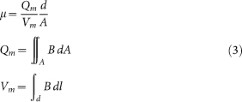With H polarized along, we obtain μy, one of the permeability principal values, by substituting d = ay, A = ax × az and B = By into (3).

To compute μx, the remaining transverse component, one replaces the incident field Ex(z) with Ey(z) and utilizes the appropriate fields and dimensions in (3). To compute the normal component μz, however, an additional subtle change has to be made. We begin by exciting an incident field Ey(x) of the form, which gives rise to an almost uniform H-field Hz. However, such a field still violates the periodic condition along x slightly; therefore on the x = const faces we use Floquet (phase-shifted periodic) boundary condition with the phase shift given by kxax. We then compute μz from (3), substituting d = az, A = ax × ay.

We perform field-averaging retrievals across the 8 MHz–16 MHz frequency range and compute the transverse and normal components of μ for both the 1- and 3-layers configurations. We then fit each retrieved permeability to a Lorentzian curve defined as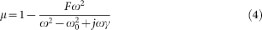where F is a constant representing the oscillator's strength, ω0 = 2πf is the angular resonance frequency and y = ω0/2Q. The resulting fitted parameters of the 1-layer MM are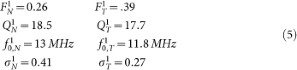and the parameters fitted from the 3-Layer MM retrieval were calculated to be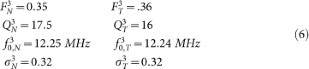Here we have used the superscripts 1 and 3 to distinguish between the 1- and 3-layer slabs, respectively and the subscripts N and T to distinguish between the normal and transverse permeability components, respectively. Not surprisingly, comparing the fitted parameters for the 1- and 3-layer MM suggests that as more layers are added the metamaterial behaves in a more isotropic fashion.

We note that the Lorentzian parameters provide a quality fit for the complex permeability curve only in the vicinity of the fundamental magnetic resonance studied. Good quality of fit is maintained at least through the frequency where Re(μ) crosses zero (roughly 16 MHz), that is, in the entire frequency band of interest.

### Maximum transducer power gain calculations

Before we conduct WPT measurements with the non-resonant coils setup, we perform a calibration that moves reference planes of a VNA to the end of the cables that are connected to the coils (see Figure 7). This enables us to retrieve the direct coil-to-coil transmission efficiency. In Ref. 23. Pozar describes a suitable metric called the Maximum Transducer Power Gain,, which is the gain that would be achieved if a lossless matching network was inserted between the NA's reference planes and the non-resonant loops. Pozar definesin terms of only S parameters; here we summarize the calculations outlined in Ref. 23.

Transducer Power Gain GT is the ratio of power delivered to the load, PL, to the power available from the source, ps: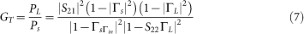where ΓL = (ZLZ0)/(ZL + Z0) is the reflection coefficient seen looking toward the load, ΓS = (ZSZ0)/(ZS + Z0) is the reflection coefficient seen looking toward the source and Γin is the reflection coefficient seen looking toward the input of the two port network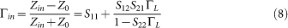where Zin is the impedance seen looking into port 1 of the terminated network. Similarly, Γout is the reflection coefficient seen looking into port 2 of the network when port 1 is terminated by ZS: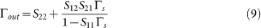The Maximum Transducer Power Gain,, occurs whenand. In the general case with a bilateral two port network, Γin is affected by Γout and vice versa, so that the input and output must be matched simultaneously. Equatingandwith the RHS of (8) and (9), respectively, yields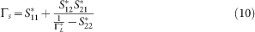where Δ = S11S22S12S21. Substituting (10) into (9) and rearranging the terms results in the quadratic equation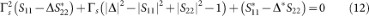yielding the solutions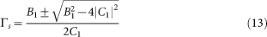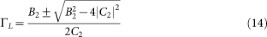where B1 = 1 + |S11|2 − |S22|2 − |Δ|2,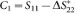, B2 = 1 + |S22|2 − |S11|2 − |Δ|2 and. To convert the recorded S-parameters to, then, one must first calculate (in order) Δ, C1, C2, B1, B2, ΓL, ΓS and Γin and use these parameters in (12).

## References

1. Pendry, J. B. Negative refraction makes a perfect lens. Phys. Rev. Lett. 85, 3966–3969 (2000).

2. Melville, D. O. S., Blaikie, R. J. & Wolf, C. R. Submicron imaging with a planar silver lens. Appl. Phys. Lett. 84, 4403–4405 (2004).

3. Fang, N., Lee, H., Sun, C. & Zhang, X. Sub-diffraction-limited optical imaging with a silver superlens. Science 308, 534–537 (2005).

4. Taubner, T., Korobkin, D., Urzhumov, Y., Shvets, G. & Hillenbrand, R. Near-field microscopy through a SiC superlens. Science 313, 1595–1595 (2006).

5. Wiltshire, M. C. K. et al. Microstructured magnetic materials for RF flux guides in magnetic resonance imaging. Science 291, 849–851 (2001).

6. Wiltshire, M. C. K., Hajnal, J. V., Pendry, J. B., Edwards, D. J. & Stevens, C. J. Metamaterial endoscope for magnetic field transfer: near field imaging with magnetic wires. Opt. Express 11, 709–715 (2003).

7. Bingnan, W. et al. Wireless power transfer with metamaterials. Paper presented at Proceedings of the 5th European Conference on Antennas and Propagation (EUCAP), Rome. (2011 April 11–15).

8. Kurs, A. et al. Wireless power transfer via strongly coupled magnetic resonances. Science 317, 83–86 (2007).

9. Urzhumov, Y. & Smith, D. R. Metamaterial-enhanced coupling between magnetic dipoles for efficient wireless power transfer. Phys. Rev. B 83, 205114 (2011).

10. Merlin, R. Radiationless electromagnetic interference: evanescent-field lenses and perfect focusing. Science 317, 927–929 (2007).

11. Grbic, A., Jiang, L. & Merlin, R. Near-field plates: subdiffraction focusing with patterned surfaces. Science 320, 511–513 (2008).

12. Grbic, A., Merlin, R., Thomas, E. M. & Imani, M. F. Near-field plates: Metamaterial surfaces/arrays for subwavelength focusing and probing. Proc. IEEE 99, 1806–1815 (2011).

13. Merlin, R. Analytical solution of the almost-perfect-lens problem. Appl. Phys. Lett. 84, 1290–1292 (2004).

14. Wang, B. N. et al. Experiments on wireless power transfer with metamaterials. Appl. Phys. Lett. 98, 254101 (2011).

15. Freire, M. J., Marques, R. & Jelinek, L. Experimental demonstration of a μ = −1 metamaterial lens for magnetic resonance imaging. Appl. Phys. Lett. 93, 231108 (2008).

16. Freire, M. J., Jelinek, L., Marques, R. & Lapine, M. On the applications of μr = −1 metamaterial lenses for magnetic resonance imaging. J. Magn. Reson. 203, 81–90 (2010).

17. Huang, D., Urzhumov, Y., Smith, D. R., Teo, K. H. & Zhang, J. Y. Magnetic superlens-enhanced inductive coupling for wireless power transfer. J. Appl. Phys. 111, 064902 (2012).

18. Chen, W.-C., Bingham, C. M., Mak, K. M., Caira, N. W. & Padilla, W. J. Extremely subwavelength planar magnetic metamaterials. Phys. Rev. B 85, 201104 (2012).

19. Shamonina, E. & Solymar, L. Magneto-inductive waves supported by metamaterial elements: components for a one-dimensional waveguide. J. Phys. D: Appl. Phys. 37, 362 (2004).

20. Smith, D., Vier, D., Koschny, T. & Soukoulis, C. Electromagnetic parameter retrieval from inhomogeneous metamaterials. Phys. Rev. E 71, 036617 (2005).

21. Chen, X., Grzegorczyk, T. M., Wu, B.-I., Pacheco Jr, J. & Kong, J. A. Robust method to retrieve the constitutive effective parameters of metamaterials. Phys. Rev. E 70, 016608 (2004).

22. Urzhumov, Y. A. & Shvets, G. Optical magnetism and negative refraction in plasmonic metamaterials. Solid State Commun. 146, 208–220 (2008).

23. Pozar, D. M. Microwave engineering (J. Wiley, Hoboken, NJ, 2005).

## Acknowledgements

This work was financially supported by the Toyota Research Institute of North America.

## Author information

Authors

### Contributions

D.R.S. and Y.U. developed quantitative models for superlens-assisted power transfer and assisted in the analysis of simulations and experiments, Y.U., D.H. and K.S. developed the simulation algorithms, G.L. and K.S. performed numerical calculations, G.L. and P.S. designed PCB layouts, P.S., M.R. and J.E. constructed the metamaterial lens, M.R., J.E., P.S., T.N. and J.S.L. designed and conducted the experiments and J.S.L. developed the project. All authors participated in discussing the results and writing the manuscript.

## Ethics declarations

### Competing interests

The authors declare no competing financial interests.

## Rights and permissions

Reprints and Permissions

Lipworth, G., Ensworth, J., Seetharam, K. et al. Magnetic Metamaterial Superlens for Increased Range Wireless Power Transfer. Sci Rep 4, 3642 (2014). https://doi.org/10.1038/srep03642

• Accepted:

• Published:

• ### Wireless power transfer system rigid to tissue characteristics using metamaterial inspired geometry for biomedical implant applications

• Ramesh K. Pokharel
• , Shimaa Alshhawy
• , Kuniaki Yoshitomi
•  & Costas Sarris

Scientific Reports (2021)

• ### A novel approach to Fabry–Pérot-resonance-based lens and demonstrating deep-subwavelength imaging

• Md. Anzan-Uz-Zaman
• , Kyungjun Song
• , Duck-Gyu Lee
•  & Shin Hur

Scientific Reports (2020)

• ### Wide-angle metamaterial absorber with highly insensitive absorption for TE and TM modes

• Majid Amiri
• , Negin Shariati
• , Justin Lipman
•  & Mehran Abolhasan

Scientific Reports (2020)

• ### A compact wideband metamaterial absorber for Ku band applications

• Chetan Barde
• , Arvind Choubey
• , Rashmi Sinha
• , Santosh Kumar Mahto
•  & Prakash Ranjan

Journal of Materials Science: Materials in Electronics (2020)

• ### Calculation and analysis of near-field magnetic spiral metamaterials for MCR-WPT application

• Xiutao Huang
• , Conghui Lu
•  & Minghai Liu

Applied Physics A (2020)